# 4. American Airlines flights from Dallas to Chicago are on time 80% of the time. Suppose...

4. American Airlines flights from Dallas to Chicago are on time 80% of the time. Suppose 15 flights are randomly selected, and the number of on-time flights is recorded.

a. Is this a binomial experiment? Explain.

b. Find the probability that exactly 10 flights are on time.

c. Find the probability that fewer than 10 flights are on time.

d. Find the probability that at least 10 flights are on time.

e. Find the mean and the standard deviation of flights that are on time. If 13 flights are on time, would that be significant?

This Homework Help Question: "4. American Airlines flights from Dallas to Chicago are on time 80% of the time. Suppose..." No answers yet.

We need 10 more requests to produce the answer to this homework help question. Share with your friends to get the answer faster!

0 /10 have requested the answer to this homework help question.

Once 10 people have made a request, the answer to this question will be available in 1-2 days.
All students who have requested the answer will be notified once they are available.
##### Add Answer to: 4. American Airlines flights from Dallas to Chicago are on time 80% of the time. Suppose...
Similar Homework Help Questions
• ### 4. According to flightstats.com, American Airline flights from Dallas to Chicago are on time 80% of...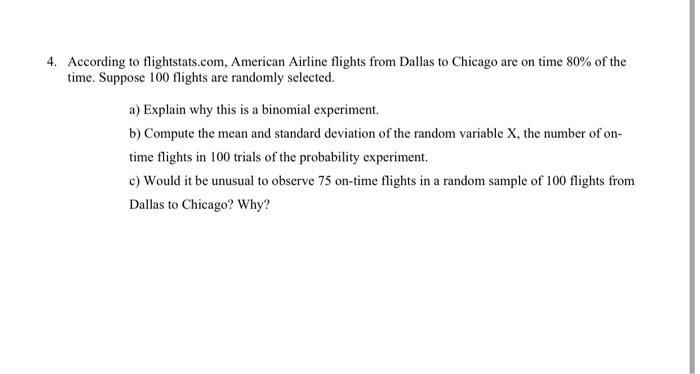4. According to flightstats.com, American Airline flights from Dallas to Chicago are on time 80% of the time. Suppose 100 flights are randomly selected. a) Explain why this is a binomial experiment. b) Compute the mean and standard deviation of the random variable X, the number of on- time flights in 100 trials of the probability experiment. c) Would it be unusual to observe 75 on-time flights in a random sample of 100 flights from Dallas to Chicago? Why?

• ### 3. According to flightstats.com, American Airlines flight from Orlando to Los Angeles is on time 60%...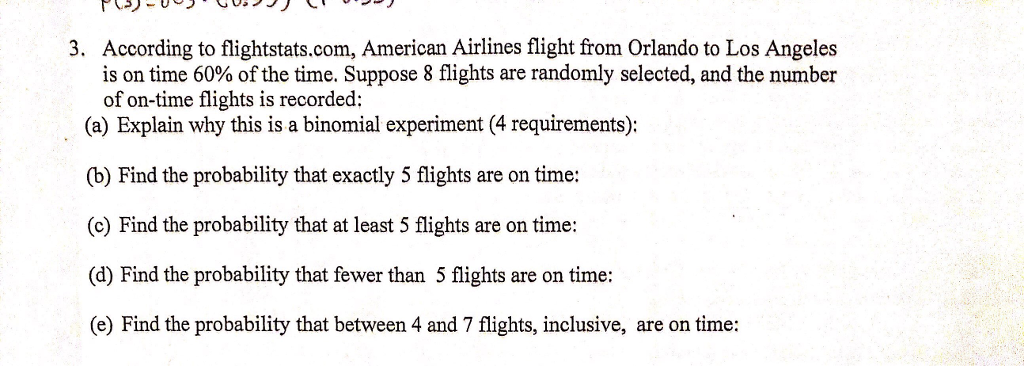3. According to flightstats.com, American Airlines flight from Orlando to Los Angeles is on time 60% of the time. Suppose 8 flights are randomly selected, and the number of on-time flights is recorded: (a) Explain why this is a binomial experiment (4 requirements): (b) Find the probability that exactly 5 flights are on time: (c) Find the probability that at least 5 flights are on time: (d) Find the probability that fewer than 5 flights are on time: (e) Find...

• ### According to an​ airline, flights on a certain route are on time 80​% of the time....

According to an​ airline, flights on a certain route are on time 80​% of the time. Suppose 24 flights are randomly selected and the number of​ on-time flights is recorded. ​(a) Explain why this is a binomial experiment. ​(b) Find and interpret the probability that exactly 16 flights are on time. ​(c) Find and interpret the probability that fewer than 16 flights are on time. ​(d) Find and interpret the probability that at least 16 flights are on time. ​(e)...

• ### According to an​ airline, flights on a certain route are on time 85​% of the time....

According to an​ airline, flights on a certain route are on time 85​% of the time. Suppose 20 flights are randomly selected and the number of​ on-time flights is recorded. ​(a) Explain why this is a binomial experiment. ​(b) Find and interpret the probability that exactly 13 flights are on time. ​(c) Find and interpret the probability that fewer than 13 flights are on time. ​(d) Find and interpret the probability that at least 13 flights are on time. ​(e)...

• ### According to an airline, flights on a certain route are on time 80% of the time....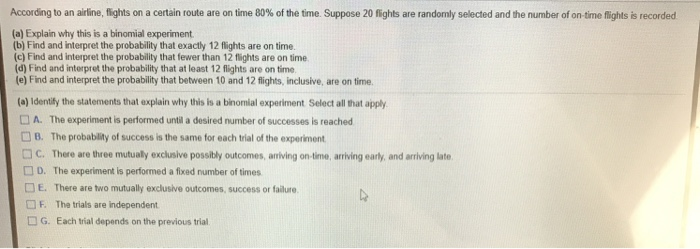According to an airline, flights on a certain route are on time 80% of the time. Suppose 20 fights are randomly selected and the number of on-time flights is recorded (a) Explain why this is a binomial experiment (b) Find and interpret the probability that exactly 12 flights are on time. (c) Find and interpret the probability that fewer than 12 flights are on time (d) Find and interpret the probability that at least 12 flights are on time (e)...

• ### A certain flight arrives on time 80 percent of the time. Suppose 174 flights are randomly...

A certain flight arrives on time 80 percent of the time. Suppose 174 flights are randomly selected. Use the normal approximation to the binomial to approximate the probability that ​(a) exactly 148 flights are on time. ​(b) at least 148 flights are on time. ​(c) fewer than 133 flights are on time. ​(d) between 133 and 150​, inclusive are on time.

• ### Suppose flight AA380 (from NYC to Chicago) arrives on time 87% of the time. Suppose 120...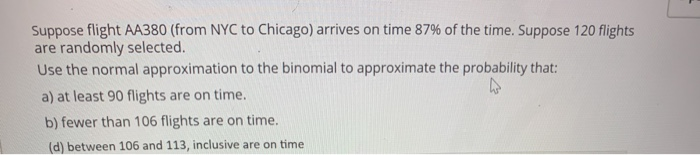Suppose flight AA380 (from NYC to Chicago) arrives on time 87% of the time. Suppose 120 flights are randomly selected. Use the normal approximation to the binomial to approximate the probability that: a) at least 90 flights are on time. b) fewer than 106 flights are on time. (d) between 106 and 113, inclusive are on time

• ### According to an airline, flights on a certain route are on time 75% ofthe time Suppose...According to an airline, flights on a certain route are on time 75% ofthe time Suppose 17 ights are rand nly selected and then mber of ontmeightsbea ded (a) Explain why this is a binomial experiment. (b) Find and interpret the probability that exactly 10 flights are on time (c) Find and interpret the probability that fewer than 10 flights are on time (d) Find and interpret the probability that at least 10 flights are on time (e) Find and...

• ### Question Help According to an airline, flights on a certain route are on time 75% of...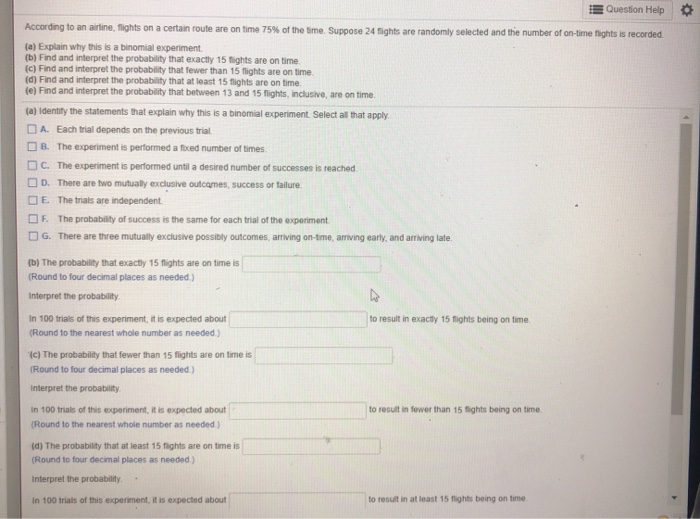Question Help According to an airline, flights on a certain route are on time 75% of the time. Suppose 24 flights are randomly selected and the number of on-time flights is recorded. (a) Explain why this is a binomial experiment. (b) Find and interpret the probability that exactly 15 lights are on time (c) Find and interpret the probability that fewer than 15 flights are on time (d) Find and interpret the probability that at least 15 fights are on...

• ### *Problem #36) The problem numbers below correspond to problems in the textbook for section 6.2 (pg. 340). Use the scenar...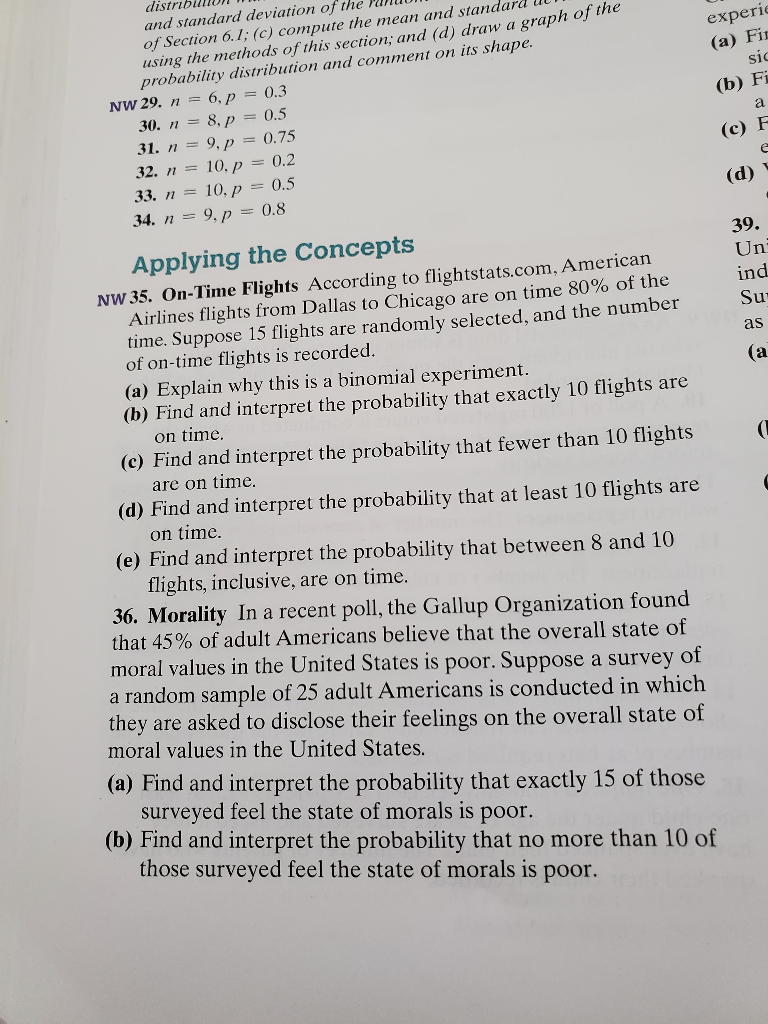*Problem #36) The problem numbers below correspond to problems in the textbook for section 6.2 (pg. 340). Use the scenario given in the textbook problem to answer the questions given here. In other words, replace parts (a), (b), etc. from the book with the questions below. Please show the probabilities necessary to support all of your answers, particularly when asked whether a particular result is unusual. Problem #36) (a) Identify the properties of this binomial distribution: What does a "success"...

Free Homework App